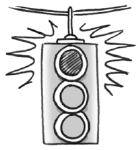### Home > AC > Chapter 3 > Lesson 3.2.2 > Problem3-85

3-85.For each equation, a possible solution is given. Check to see if the given solution is correct.

1. If $3x+7=x-1$, then does $x=-4$?

Substitute the possible answer for $x$.

$3(-4)+7=(-4)-1$

If the numbers on both sides of the equation sign are the same once simplified, then the possible answer is correct.

$-12+7=-5$
$-5=-5$

Yes

2. If $-2x-4=-4x+3$, then does $x=3$?

Follow the steps in part (a).

No

3. If $-3x+5+5x-1=0$, then does $x=2$?

Follow the steps in part (a).

4. If $-(x-1)=4x-5-3x$, then does $x=3$?

How does the negative in front of the parentheses change what is inside?

Follow the steps in part (a).